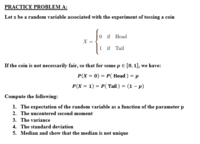# Computing the median of a random variable

#### Jingle Bells

##### New memberHello i am doing the following practice problem and I am stuck on part 5. I tried to compute the median by taking the highest number in my domain and subtracting the lowest number in my domain then dividing it by 2. This resulted in an solution of 1/2. However I am completely confused because since i solved for a median how can i even show its not unique, because i only got one singular value for the median.

#### Jomo

##### Elite Member
What does the median mean? How do you calculate it?